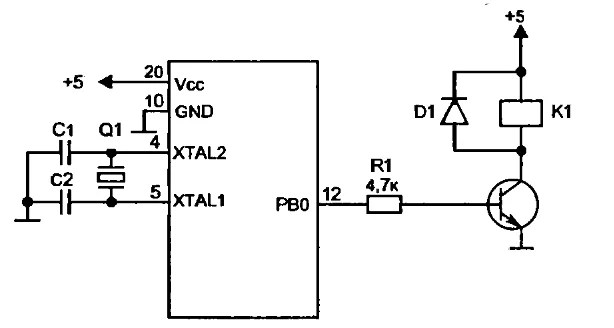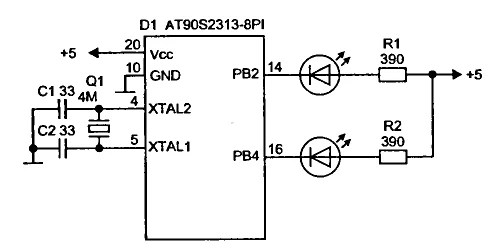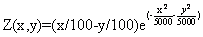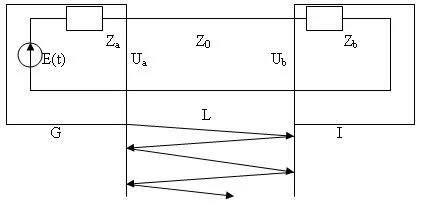## Control 7 segment LED displays with AVRLED displays are nothing more than sets of Light Emitting Diodes. The difference is that they have different shapes in order to display specific information. So driving LED displays is the same as regular LEDs. This is simple connection when there are enough of microcontroller Pins. But if you want to connect more displays you will need more microcontroller pins than it can give you. Then you need to make more advanced circuit with dynamic control.

## Drive relay with AVR microcontrollerTo drive relay you need more than 20mA – the current can one pin drive. This is why you cannot connect relay directly to microcontrollers pin. To drive relay you need to connect simple amplifier made of one transistor. One important part of this circuit is the diode, which protects circuit from induction caused when switching relay.

## Drive LED or Opto-isolators with AVRThis might be seem very simple to many of you, but I still get questions about simple microcontroller interfacing. So I will put a thread of notes about interfacing AVR microcontrollers to devices like LED’s, relays, I2C, etc. As you might know Diodes require pretty small current. This current depends on diode type and can be from 3mA up to 20mA and more. Working voltage is from 1.5 to 4V. One AVR pin can sink up to 20mA of current; it is convenient to connect diode directly to it with current limiting resistor. Never connect diode to pin without resistor – you may damage your AVR as your current may exceed the 20mA limit!

## K-Type Thermocouples reviewedA thermocouple is a sensor that generates an electrical potential related to the temperature. The operating principle of the sensor is base on the fact that any electrical junction between two different metals generates an electrical potential that depends on the temperature and the metals that are used. The principle applies equally well if three metals are used. In that case, there are two junctions in series, and the net potential results from the series addition of the two individual potentials. For example, if a copper iron junction is in series with an iron-tin junction, the net potential is the same as for a copper-tin junction. However, that is only true if both junctions are at the same temperature. The K-Type thermocouple is usually made of Chromel (+) and Alumel(-). The voltage generated from this sensor is 4mV/100Â°C. The max temperature can thermocouple withstand is 1000Â°C without any damage. How ever thermocouples have a drawback. It is because connecting thermocouple to circuit creates addition junctions between different metals who generate additional potentials. Generally speaking measuring thermocouple potential output is measuring not direct function of absolute temperature, but a difference between temperature at measurement point and temperature at the connection point.…

## Gauss-Zeidel optimization routinesThis is simplest optimization routines. Using this algorithm optimization parameters are changed separately in each step. Only one parameter can be changed in one step while other are helt as constants. Xk+1=Xk+ΔXk , k=0,1,2,… ΔXk step of parameter Xk. Parameter is changed until function growth is noticed, and then next parameter follows and so on. After cycle with all parameters is completed, then step is changed to half of its value and repeat cycle again. Optimal point searching ends when there is no function increase and last point is held as optimal point. Lets see how it works with following function: Its plot: Using MATLAB script we get results bellow. In each picture start coordinates are different. Start coordinates. x=150; y=200; Start coordinates x=50; y=150; Other example Start coordinates x=10; y=10; Start coordinates x=100; y=200; Third example Start coordinates x=10; y=10; Start coordinates x=50; y=200; Matlab script: close all; clear all; clc; [X,Y] = meshgrid(-100:1:100, -100:1:100); Z =3*exp(-((X.^2)/78000) -((Y.^2)/20000))-5*exp(-(((X+31).^2)/123) -(((Y+20).^2)/5000)); xx=100; yy=100; contour3(Z,20); hold on; % figure(2); mesh(Z); % figure % plot3(X,Y,Z) X=70; Y=100; X1=0;Y1=0; X0=X; Y0=Y; z=160; figure(1); plot(X,Y,’r*’), hold on; X=X-xx; Y=Y-yy; TT1 =3*exp(-((X.^2)/78000) -((Y.^2)/20000))-5*exp(-(((X+31).^2)/123) -(((Y+20).^2)/5053)); for i=1:50 z=z/2; X=X-z; Z2 =3*exp(-((X.^2)/78000) -((Y.^2)/20000))-5*exp(-(((X+31).^2)/123) -(((Y+20).^2)/5053)); if Z2>=TT1 TT=Z2; X1=X;…

## Impulse signal distortion in transmission lineToday most electronic equipment consists of signal generators and processing units. These units are connected with transmission lines. These lines have big influence in signal distortions. On these lines depends transmition lines stability. Letâ€™s see how transmition lines affect transmitted signals. (G- signal generator; Za– output impedance; I- signal receiver Zb– input impedance; L- transmission line length; Z0– Line impedance. When line is tuned and without losses then input voltage: Ub(t) = ZbE(t-t)/(Za + Zb) E(t)- generators signal amplitude; t– signal delay in line. t =l/v ; l- line lenght; v- signal speed. If line is not tuned up, then there are distortions in line because of reflections in line: If signal E(t) is step function: Then signal in line exit in discrete time moments will be: U(0) = p; U(t) = p*(1+pb) U(2t) = p*(1+pb+pa*pb) U(3t) = p*(1+pb+pa*pb+pa*pb^2); U(4t) = p*(1+pb+pa*pb+pa*pb^2+pa^2*pb^2); U(5t) = p*(1+pb+pa*pb+pa*pb^2+pa^2*pb^2+pa^2*pb^3) â€¦ where Depending on reflectance coefficients and their signs distortions can differentiate or integrate: Real model using MathCAD was implemented to see how signal looks like on exit depending on parameters. Below you see used algorithm structure used in modeling: Algorithm Part 1 Algorithm Part 2 One of results using trapezoid signal: In exit we…